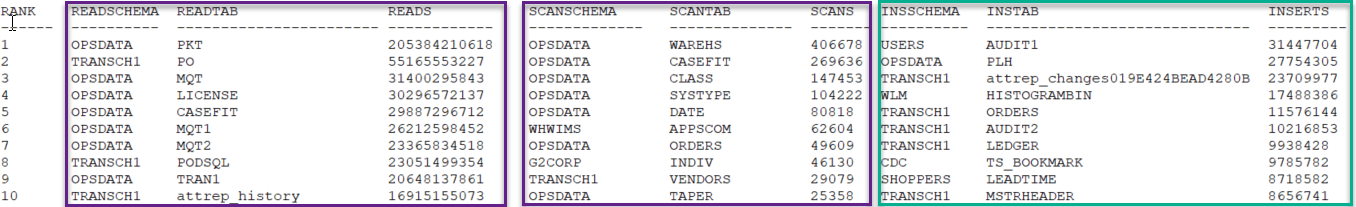Select Page

# Raghu On Tech

Pro tips on managing data at scale

# DB2 Finding Top 10 Most Active Tables ?

by | Feb 29, 2020 | 3 comments

### Problem:

How would you generate a report of the top 10 most active tables of your DB2 database ? To be more precise how would you generate a list of top 10 tables that are being Read/Inserted/Updated/Deleted and also against which most table scans are happening ?

### Solution:

Use the following SQL to generate a list of top 10 tables by Reads/Table Scans/Inserts/Updates/Deletes.

```with topins(insrank, insschema, instab, inserts) as (
select * from (select row_number() over(order by sum(rows_inserted) desc) as ranking, trim(tabschema), trim(tabname), sum(rows_inserted)
from table(mon_get_table('','',-2)) as tb group by tabschema, tabname) where ranking<=10),
select * from (select row_number() over(order by sum(rows_read) desc) as ranking, trim(tabschema), trim(tabname), sum(rows_read)
from table(mon_get_table('','',-2)) as tb group by tabschema, tabname) where ranking<=10),
topupd(updrank, updschema, updtab, updates) as (
select * from (select row_number() over(order by sum(rows_updated) desc) as ranking, trim(tabschema), trim(tabname), sum(rows_updated)
from table(mon_get_table('','',-2)) as tb group by tabschema, tabname) where ranking<=10),
topdel(delrank, delschema, deltab, deletes) as (
select * from (select row_number() over(order by sum(rows_deleted) desc) as ranking, trim(tabschema), trim(tabname), sum(rows_deleted)
from table(mon_get_table('','',-2)) as tb group by tabschema, tabname) where ranking<=10),
topscans(scanrank, scanschema, scantab, scans) as (
select * from (select row_number() over(order by sum(table_scans) desc) as ranking, trim(tabschema), trim(tabname), sum(table_scans)
from table(mon_get_table('','',-2)) as tb group by tabschema, tabname) where ranking<=10)

substr(scanschema,1,20) as scanschema, substr(scantab,1,30) as scantab, s.scans,
substr(insschema,1,20) as insschema, substr(instab,1,30) as instab, inserts,
substr(updschema,1,20) as updschema, substr(updtab,1,30) as updtab, u.updates,
substr(delschema,1,20) as delschema, substr(deltab,1,30) as deltab, d.deletes
from topins i, topread r, topupd u, topdel d, topscans s
order by insrank
with ur;```

Running the above SQL will provide you with an output that looks something like below. Output is truncated as its a wide row and could not fit my putty session. I usually run this query through my Data studio for better visuals/optics.As you can see from the above output, you are getting a nice view of tables ranked by number of rows read, table scans performed rows inserted, rows updated, rows deleted etc all this info with single query. This query takes advantage of common table expressions along with OLAP functions to make it look nice and simple. With out OLAP functions you are looking at scalar subqueries which can be clumsy at times.

In the above screen shot, I divided the output into three sections, first section are the list of tables ranked by ROWS_READ, second section are the list of tables ranked by TABLE SCANS and third section are the list of tables ranked by INSERT activity. This kind of information is invaluable especially when you collect this information and store it over a period of time. You can run reports and identify changes in workload and proactively act before it becomes a noticeable issue. For e.g. if you notice that a new table showed up in your top 10 list of table scans, you know there is something wrong and you will follow up to determine if application deployed a new code or find that offending query whose access path may have changed recently.

Once, you identify the tables that are driving the most READ IO, you could work out a plan to identify the queries driving this IO. One way to do this is enable an activity event monitor and identify SQL accessing the tables at the top of your list. Other ways include usage of USAGE LISTS or querying MON_GET_PKG_CACHE_STMT.

Hope you learned something by reading this short blog post. Leave comments on what other SQL you use to get some common metrics against your DB2 database ?

Follows

1.Thanks Raghu, great query! I made a simple variant to find the top 100 inactive.

with topins(insrank, insschema, instab, inserts) as (
select * from (select row_number() over(order by sum(rows_inserted)) as ranking, trim(tabschema), trim(tabname), sum(rows_inserted)
from table(mon_get_table(”,”,-2)) as tb where tabschema not in (‘SYSIBM’) group by tabschema, tabname) where ranking<=100 ),
select * from (select row_number() over(order by sum(rows_read)) as ranking, trim(tabschema), trim(tabname), sum(rows_read)
from table(mon_get_table('','',-2)) as tb where tabschema not in ('SYSIBM') group by tabschema, tabname) where ranking<=100 ),
topupd(updrank, updschema, updtab, updates) as (
select * from (select row_number() over(order by sum(rows_updated)) as ranking, trim(tabschema), trim(tabname), sum(rows_updated)
from table(mon_get_table('','',-2)) as tb where tabschema not in ('SYSIBM') group by tabschema, tabname) where ranking<=100 ),
topdel(delrank, delschema, deltab, deletes) as (
select * from (select row_number() over(order by sum(rows_deleted)) as ranking, trim(tabschema), trim(tabname), sum(rows_deleted)
from table(mon_get_table('','',-2)) as tb where tabschema not in ('SYSIBM') group by tabschema, tabname) where ranking<=100 ),
topscans(scanrank, scanschema, scantab, scans) as (
select * from (select row_number() over(order by sum(table_scans)) as ranking, trim(tabschema), trim(tabname), sum(table_scans)
from table(mon_get_table('','',-2)) as tb where tabschema not in ('SYSIBM') group by tabschema, tabname) where ranking<=100 )

substr(scanschema,1,20) as scanschema, substr(scantab,1,30) as scantab, s.scans,
substr(insschema,1,20) as insschema, substr(instab,1,30) as instab, inserts,
substr(updschema,1,20) as updschema, substr(updtab,1,30) as updtab, u.updates,
substr(delschema,1,20) as delschema, substr(deltab,1,30) as deltab, d.deletes
from topins i, topread r, topupd u, topdel d, topscans s
order by insrank
with ur;

2.db2pd (one liner) is used in my practice to obtain “hot” SUID and TableScans

3.Good effort for the community, Congrats!